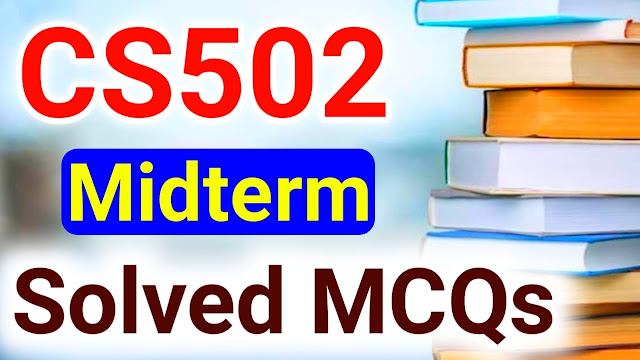# CS502 Midterm MCQs

CS502 Midterm Solved MCQs: In this post students, you will read which you search on google that CS502 Midterm MCQs are very informative and helpful MCQs for those students who want to prepare their exams but they could not find the right place from where they can easily find these . But we are always here to help our VU students by making helpful study solutions so that our students can easily search on google and they can find these CS502 Midterm MCQs without any cost because many websites on the internet demand money to find any book study solutions but students here you will find any book solutions free of cost but we need your support only to your our this post with more students. This is the CS502 - Fundamentals of Algorithms MCQs for the midterm. Students you read then you like it we hope you will also try to share fundamentals of algorithms MCQs with other students. Also, Read CS502 Quiz 1 Solution.

### CS502 - Fundamentals of Algorithms MCQs

 Post Title CS502 Midterm MCQs Book Code CS502 Degree BSIT/BSCS/Other University Virtual Univerity of Pakistan CS502 Quiz 1/ Quiz 2/ Quiz 3 CS502 Midterm MCQs Mid/Final CS502 Midterm Solved MCQs Also, Read CS502 Quiz 1 MCQs Also, Read CS502 Quiz 1 2022

## CS502 Midterm Solved MCQs

PUSH and POP terminologies are used in …………..(CS502)

Stack

Double-ended queue

Circular queue

Queue

If we have an equation Bn2+7f+n+5f+6 then n is large ………….term will be much larger than the n term and will dominate the running time.  (CS502)

g(n)^2

n^2

f(g(n)

f(n)

RAM is an idealized machine with …………large random access memory.  (CS502)

Average

Finite

Infinite

Small

To find maximal points in brute force algorithm each point of the space is compared against ………….of that space.  (CS502)

One other point

Most of the other points

All other points

Few other points

If input n is odd then the median will be …….. (CS502)

(n+1)/2

N+2

n/2

(n-1)/2

An efficient algorithm requires less computational …………..(CS502)

Memory

Notation

Running time

Memory and running time

In an asymptotical analysis of n(n-3) and 4n n as n becomes large the dominant (fastest growing) term is some constant times …………..(CS502)

N+1

N*n

N-1

N

In the selection problem, the sleeve technique works in …………(CS502)

Constant time

Phases

One complete go

Nonrecursive manner

Best ………helps in minimizing algorithm cost.  (CS502)

Analysis

Input

Output

Design

The result of asymptotical analysis of n(n-3) and 4n+n is that ……………(CS502)

n(n-1) is asymptotically greater

Both are asymptotically not equivalent

n(n-1) is asymptotically less

Both are asymptotically equivalent

An algorithm is a mathematical entity that is independent of ……………..(CS502)

Programming language

Programming language compiler and machine

Compiler and programming language

Machine and programming language

The definition of theta-notation relies on proving ……..asymptotic bound.  (CS502)

Both lower and upper

Upper

One

Lower

In order to say anything meaningful about our algorithms, it will be important for us to settle on a …………..(CS502)

C++ program

Java program

Mathematical model of computation

Pseudo program

Asymptotic growth of plane sweep algorithm for 2-D maximum problem is …………(CS502)

8(logn)

8(nlogn)

8(n^2)

8(n)

Which symbol is used for omega notation?  (CS502)

(@)

(0)

(8)

(Ω)

In sleeve technique, we solve the problem …………..(CS502)

Using brute force technique

In a recursive manner

Non recursively

Using merge sort algorithm

Which of the following is calculated with big omega notation?  (CS502)

Upper bounds

Both upper and lower bounds

Medium bounds

Lower bounds

If the indices passed to merge sort algorithm are ……….then this means that there is only one element to sort.  (CS502)

Large

Small

Equal

Not equal

Pseudocode of algorithms are to be read by …………(CS502)

Compiler

RAM

Computer

People

B(1) means …………………(CS502)

That it is used for large n

That if used for small n

A constant amount of time

Time can not be calculated

For …………..values of n, any algorithm is fast enough.  (CS502)

Large

Small

Infinity

Medium

In brute force algorithm, we measure running time T(n) based on ……………(CS502)

Best case time and starting case time

Worst-case time and best case time

Average case time and best case time

Worst-case time and average-case time

In process of ……………ends when you are left with such tiny pieces remaining that it is trivial to solve them.  (CS502)

Plan-sweep

Brute-force

Divide and conquer

Axis sweep

The brute force algorithm for 2D maximum runs in order O(...............) time.  (CS502)

N3

n(logn)

N

Nn

In merge sort algorithm to merge two lists of size n/2 to a list of size n takes …………time.  (CS502)

Theta log(n)

Theta (n)

Theta log2 (n)

Theta nlog (n)

Asymptotic notations are used to describe ………..of an algorithm.  (CS502)

Size

Compile-time

Length

Running time

The worst-case running time of quicksort is ………..in order to sort an array of n.  (CS502)

O(n2)

O(n)

O(nlogn)

O(logn)

Two functions 4n2 and (Bn2+2n-3) are …………..(CS502)

Not equivalent

Related to power functions

Asymptotically equivalent

Hard to calculateCS502 Midterm MCQs